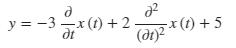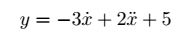# Pretty print derivative in Newton notation with dot?

Is there any way to get the pretty printer to produce Newton's notation? - ie. a single dot centred over the variable for first derivative with respect to time, 2 dots for second derivative etc.

Example:

t, y = var('t, y')
x = function('x')(t)
pretty_print(y == 2*diff(diff(x,t),t) - 3 * diff(x,t) + 5)


gives:but I'd like to see:edit retag close merge delete

• an example of input that one can paste in a fresh Sage session
• the current output
• the desired output

Sort by » oldest newest most voted

This gets requested from time to time and we should support that!

Not sure the pretty printer can do that, but there are workarounds.

One of them consists in defining "x dot" and "x dot dot" functions, and to substitute them in the equation.

sage: t, y = SR.var('t, y')
sage: x = function('x')(t)
sage: xdot = function('xdot', latex_name=r'\dot x')
sage: xddot = function('xddot', latex_name=r'\ddot x')
sage: de = y == 2*diff(diff(x, t), t) - 3 * diff(x, t) + 5
sage: dde = de.subs({diff(x, t): xdot(t), diff(diff(x, t), t): xddot(t)})
sage: view(dde)


$$y = 2 \ddot x\left(t\right) - 3 \dot x\left(t\right) + 5$$

Even better, when defining a function, you can say what its derivative is.

Using that, start by defining the furthest derivative you'll need (here xdd for "x dot dot"), then work backwards until you define x.

Then the pretty printer gets things right without the need for subs.

sage: t, y = SR.var('t, y')
sage: xdd = function('xdd', latex_name=r'\ddot x')
sage: xd = function('xd', latex_name=r'\dot x',
....:               derivative_func=lambda self, *aa, **ka: xdd(*aa))
sage: x = function('x', derivative_func=lambda self, *aa, **ka: xd(*aa))
sage: de = y == 2*diff(diff(x(t), t), t) - 3 * diff(x(t), t) + 5
sage: view(de)


$$y = -3 \, \dot x\left(t\right) + 2 \, \ddot x\left(t\right) + 5$$

more